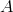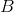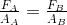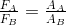Pascal’s Principle

The earth’s atmosphere exerts a pressure on all objects with which it is in contact.  Atmospheric pressure acting on a fluid is transmitted throughout that fluid.  For example, the water pressure at 100. m below the surface of a lake is 9.8 × 105 Pa.  The total pressure at that point, however, is the pressure of the water plus the pressure of the air above the water.  The pressure of the air at the surface of the water is 1.0 × 105 Pa, or 1 atm (atmosphere).  Therefore, the total pressure at 100. m below the surface of the water is 9.8 × 105 Pa + 1.0 × 105 Pa = 10.8 × 105 Pa.

This is an example of Pascal’s Principle, which states that pressure applied to a confined liquid increases the pressure throughout by the same amount.  A number of practical devices take advantage of this principle.  Hydraulic brakes, hydraulic lifts, and hydraulic presses are three useful tools that make use of Pascal’s Principle.

The sketch below is an example of a hydraulic lift.  We have a confined liquid in contact with two pistons (and) of different sizes. The pressure of the liquid on these two pisons is the same (Pascal's principle). Therefore,,

andand.

Suppose that the area of pistonis 4.0 cm2 and the area of pistonis 200. cm2.  If we place an automobile weighing 10,000 N on piston, we can lift that car by exerting a force of 200 N on piston.  This is another form of  simple machine and its ideal mechanical advantage is 50.  The ideal mechanical advantage of a hydraulic lift equals the ratio of the large piston area to the small piston area.#### Summary

• Atmospheric pressure acting on a fluid is transmitted throughout that fluid.
• Pascal’s Principle states that pressure applied to a confined liquid increases the pressure throughout by the same amount.

#### Review

1. In a hydraulic lift whose input line has a cross-sectional area of 1.00 cm2 and whose output line has a cross-sectional area of 20.0 cm2, what is the largest mass (kg) that can be lifted by an input force of 1000. N?
2. In a hydraulic lift whose IMA is 50, how far (ideally) will the output platform be lifted when the input platform is depressed 100. cm?
3. A 20.0 N force is exerted on the small piston of a hydraulic system.  The cross-sectional area of the small piston is 0.0500 m2.  What is the magnitude of the weight than can be lifted by the large piston, which has a cross-sectional area of 0.100 m2?